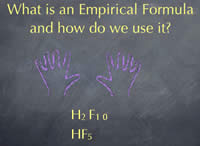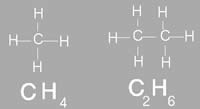# Difference between Empirical and Molecular Formula

##### Key Difference: Empirical formula of a chemical compound shows the simplest positive integer ratio of atoms of each element present in a compound. Molecular formula of a compound, also known as chemical formula, is a way of expressing the amount atoms that each element has in that particular compound.

Formulas play an important role in chemistry and physics. Though the element or molecule could be said by its common name, it does not give much information about the said element or molecule. Hence, formulas are used. Depending on the type of formula, it can provide certain types of information about the molecule that the person would have to refer it back to the periodic table. There are two types of formulas that are commonly used: Empirical and Molecular.Empirical formula of a chemical compound shows the simplest positive integer ratio of atoms of each element present in a compound. It does not refer to the compound’s isomerism, structure, or absolute number of atoms. It also does not provide the real number of atoms in a compound. For example the glucose has a molecular formula of C6H1206, so its empirical formula would be CH2O. It shows the ratio between the atoms of each element in the compound. So for ever atom of carbon and oxygen, two atoms of hydrogen are required. Empirical formula is used as standard for most ionic compounds and for macromolecules. International Union of Pure and Applied Chemistry (IUPAC) defines ‘empirical formula’ as, “Formed by juxtaposition of the atomic symbols with their appropriate subscripts to give the simplest possible formula expressing the composition of a compound.” Two different compounds can share the empirical formula. For example the molecular formula of butane is C4H8, which makes its empirical formula CH2, while the molecular formula of ethylene is C2H4, which makes its empirical formula the same as CH2. However, these are two different compounds. Empirical formula is commonly used to figure out the molecular formula of a compound.Molecular formula of a compound, also known as chemical formula, is a way of expressing the amount atoms that each element has in that particular compound. For example the molecular formula of ethanol is CH3CH2OH, which denotes that it has 6 hydrogen atoms, two carbon atoms and one oxygen atom. The placement and link of the atoms make up the compound. If the compounds were differently linked, it would make a complete different compound. The chemical formula is commonly expressed using a single line of chemical element symbols, numbers and can also include other symbols, such as parentheses, dashes, brackets, and plus (+) and minus (–) signs. The molecular formula provides the proper structure of a compound. However, it does differ from the structural formula which shows how the bonds between the atoms are connected. Molecular formula is commonly used in chemical reaction to depict chemical details or changes. Molecular mass is also helpful in order to calculate the molecular mass of an element in a compound. In addition, it can also help predict the number of ions in an compound and how many of them will be released when dissolved in water in an ionic compound. Molecular formula cannot be used to determine the exact molecular arrangement of a compound. This is because a single compound can be used to create several structural formulas, known as isomers. Isomers are compounds that have the same molecular formula but a different structural formula. The molecular formula can also help reveal all the possible isomers for a molecule.

Both of these formulas are often used in chemistry and physics depending on the need of the person.

Image Courtesy: collective.chem.cmu.edu, nyu.edu